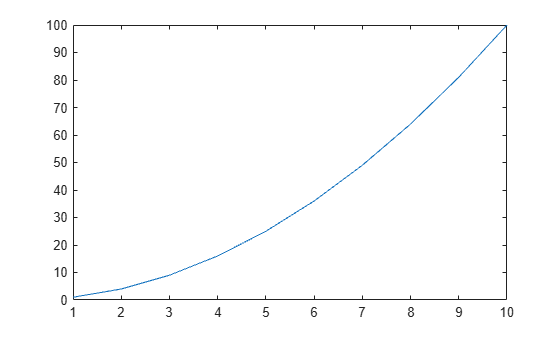# clf

## Syntax

``clf``
``clf(fig)``
``clf('reset')``
``clf(fig,'reset')``
``f = clf(___)``

## Description

example

````clf` deletes all children of the current figure that have visible handles.```

example

````clf(fig)` deletes all children of the specified figure that have visible handles.```

example

````clf('reset')` deletes all children of the current figure regardless of their handle visibility. It also resets the figure properties to their default values, except for the `Position`, `Units`, `PaperPosition`, and `PaperUnits` properties. Alternatively, you can reset the figure using the `clf reset` command without parentheses.```

example

````clf(fig,'reset')` deletes all children of the specified figure and resets its properties.```

example

````f = clf(___)` returns the figure for any of the previous syntaxes.```

## Examples

collapse all

Create a line plot. Then, set the background color of the current figure.

```x = linspace(0,2*pi); y = sin(x); plot(x,y) f = gcf; f.Color = [0 0.5 0.5];```Clear the figure using a call to `clf`. The function call deletes the plot. However, it does not affect the background color of the figure.

`clf`

Now, reset the figure properties and return the children of the figure. `clf('reset')` resets the background color to its default value.

```f = clf('reset'); f.Children```
```ans = 0x0 empty GraphicsPlaceholder array. ```

Create two figures, each with a line plot. Set the background color of the first figure.

```f1 = figure('Color','b'); plot(1:10)``````f2 = figure; plot((1:10).^2)```Clear the line plot from the first figure without resetting the background color of the figure.

`clf(f1)`

Now, reset all properties of the first figure.

`clf(f1,'reset')`

Create a figure with a white background. Then, create a surface plot.

```figure('Color','w') peaks```
``` z = 3*(1-x).^2.*exp(-(x.^2) - (y+1).^2) ... - 10*(x/5 - x.^3 - y.^5).*exp(-x.^2-y.^2) ... - 1/3*exp(-(x+1).^2 - y.^2) ```Clear the surface plot from the figure and reset all figure properties to their default values. `clf reset` resets all properties of the current figure, except for the `Position`, `Units`, `PaperPosition`, and `PaperUnits` properties.

`clf reset`

## Input Arguments

collapse all

Target figure, specified as a `Figure` object or figure number. Use `fig` to clear a specific figure, instead of the current figure.

If `fig` is a figure number, MATLAB® searches for an existing figure in which the `Number` property is equal to `fig`. By default, the `Number` property value is displayed in the title of the figure.

Example: `clf(f)` clears the figure with handle `f`.

Example: `clf(1)` clears the figure with number `1`.

collapse all

### Visible Handles

A handle is visible to `clf` if the `HandleVisibility` property of the object is set to `'on'`. When you call `clf` in the Command Window or within a callback routine, the function deletes only those objects whose `HandleVisibility` property is set to `'on'`. It does not delete objects whose `HandleVisibility` property is set to `'callback'` or `'off'`.

## Tips

• To clear the contents of a figure, you can alternatively use Clear Figure from the figure window's Edit menu. Using Clear Figure deletes all children of the figure that have visible handles.

### Properties

Introduced before R2006a